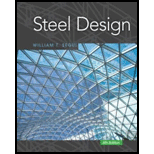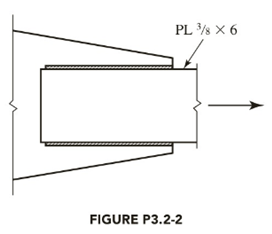# A PL 3 8 × 6 tension member is welded to a gusset plate as shown in Figure P3.2-2. The steel has a yield stress F y = 50 ksi and an ultimate tensile stress F u = 65 ksi. Assume that A e = A g and compute a . the design strength for LRFD b . the allowable strength for ASD### Steel Design (Activate Learning wi...

6th Edition
Segui + 1 other
Publisher: Cengage Learning
ISBN: 9781337094740

#### Solutions

Chapter
Section### Steel Design (Activate Learning wi...

6th Edition
Segui + 1 other
Publisher: Cengage Learning
ISBN: 9781337094740
Chapter 3, Problem 3.2.2P
Textbook Problem
44 views

## A PL 3 8 × 6 tension member is welded to a gusset plate as shown in Figure P3.2-2. The steel has a yield stress F y = 50 ksi and an ultimate tensile stress F u = 65 ksi. Assume that A e = A g and computea. the design strength for LRFDb. the allowable strength for ASDTo determine

(a)

The design strength for Load and Resistance Factor Design (LRFD).

### Explanation of Solution

Given:

A steel PL 38×6 welded tension member.

The effective area Ae=Ag.

The yield stress is 50ksi.

The tensile stress is 65ksi.

Concept Used:

Write the expression for the factored strength in yielding.

P=Φy×Pny ...... (I)

Write the expression for the factored strength in rupture.

P=Φf×Pnf ...... (II)

Here the strength of the material is P, the resistance factor for yielding is Φy, the resistance factor for rupture is Φf, the nominal strength in yielding is Pny, and the nominal strength in rupture is Pnr

The design strength of LRFD is the minimum of Equation (I) and (II).

Write the expression for the nominal strength in yielding.

Pny=Fy×Ag ...... (III)

Here, the yield strength in yielding is Fy, the gross area of the member is Ag.

Write the expression for the gross area of the member.

Ag=L×t ...... (IV)

Here, the length of the member is L, the thickness of the member is t.

Write the expression for the nominal strength in rupture.

Pnf=Fu×Ae ...... (V)

Here, the yield strength in rupture is Fu, the effective area is Ae.

Given that, Ae=Ag, hence the expression for the nominal strength in rupture is,

Pnf=Fu×Ag ...... (VI)

For the A36 steel resistance factors are,

Φy=0.90Φf=0.75

Calculation:

Calculate the gross area

To determine

(b)

The allowable strength for Allowable Strength Design (ASD).

### Still sussing out bartleby?

Check out a sample textbook solution.

See a sample solution

#### The Solution to Your Study Problems

Bartleby provides explanations to thousands of textbook problems written by our experts, many with advanced degrees!

Get Started

Find more solutions based on key concepts
Follow the steps discussed in Section 16.4 and draw the isometric view for Problem 16.13. 16.13 Problem 16.13 B...

Engineering Fundamentals: An Introduction to Engineering (MindTap Course List)

How have cloud-based data services affected the DBAs role?

Database Systems: Design, Implementation, & Management

Software suites offer three major advantages: lower cost, ease of use, and integration. (172)

Enhanced Discovering Computers 2017 (Shelly Cashman Series) (MindTap Course List)

If your motherboard supports ECC DDR3 memory, can you substitute non-ECC DDR3 memory?

A+ Guide to Hardware (Standalone Book) (MindTap Course List)